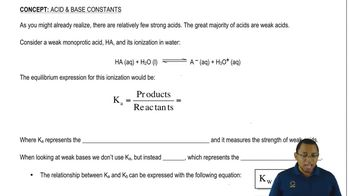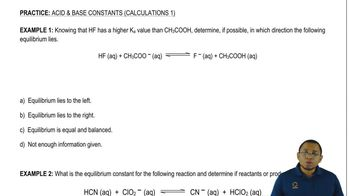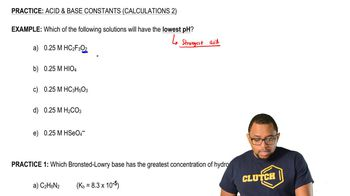Start typing, then use the up and down arrows to select an option from the list.Jules Bruno
239views
3
Now we're gonna say that K represents our acid association constant. And it basically measures how strong or weak acids are. And we're gonna say weak acids use K a week basis. Don't use K A Instead, they use K B. So this is our based association constant. What we're gonna say here is their relationship to each other. K and K B can be expressed by this formula. K w equals K a times K B. And as you realize this K w is the same k w we saw earlier in the earlier times, we saw it as K w equals h Positive times O H minus. It's the same exact kw. And we're going to say in general, the higher the K value than the stronger the acid, the stronger the acid, the greater the concentration of H plus. Now we're gonna say related to K is PK. And remember we talked about what p means. P means negative log. So PKK means negative log of K here. We're gonna say there's an inverse relationship. It's actually the lower the PK, the stronger the acid and the stronger the acid, the greater the H plus concentration now we're gonna say here is when it comes to a weak acid, we're gonna save weak ass. It tends to have k value less than one and a peek a value greater than one. Just realized we set inverse relationship. So okay, and pkr opposites. If one is up, the other one is down strong acids. Though strong acids tend tohave, Pekka is much greater than one. I mean, not PKs with K is much greater than one, and they tend to have PKK's lower than one PK that are negative. And remember, we should be able to establish a relationship between K m p k A. In the same way, we've been able to establish a relationship between H positive and pH because here we could just simply say K equals 10 to the negative p k. We could also say the same thing for PKB. P K B equals negative log of K B and therefore K B equals 10 to the negative PK. Just remember these relationships and how they affect the acidity of our compound. As long as you can remember these few quick, easy calculations, you'll be able to go from one to the other and one last thing. We could say here that the relationship from Peak to Peak A and P K B can be established by looking at this equation here by taking them negative log of K W K a n k b. We say that the equation becomes 14 equals P. K plus PKB Now that we've seen all these types of new variables and new types of equations attempt to do the practice question on the bottom here, I say If the KB of NH three is 1.76 times 10 to the negative five, what is the acid dissociation? Constant off the conjugate acid conjugate acid is a term we learned earlier. A few videos back, but here we were giving KB or has to find the acid association constant. So it's a simple conversion from one unit to another. So once you're done with that, click back on the explanation button and see how I approach this problem. Good luck, guys.01:2003:5101:3407:0901:53